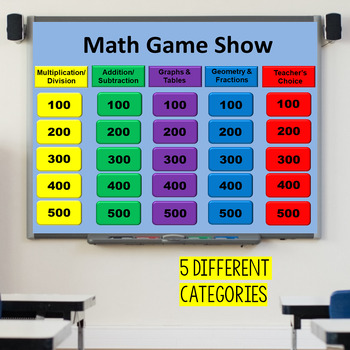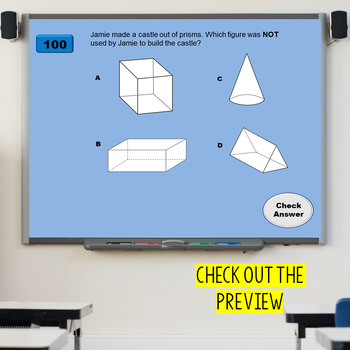# Math Review Game 3rd Grade Test Prep3rd
Subjects
Standards
Resource Type
Formats Included
• PPTX
Pages
54 pages

### Description

This is a 3rd grade test prep review game. It was made to review the 3rd grade Math STAAR test but can be used with any state assessment.

This game is like a "Jeopardy" style game. The categories include multiplication/division, addition/subtraction, graphs, generate/extend tables, and teacher's choice. The questions are all word problems that get more difficult as you increase in value.

An example of a question is listed.

Janelle has 7 pages of stickers that have 12 stickers each. Katy has 8 pages of stickers that has 9 stickers each. How many more stickers does Janelle have than Katy?

The template is editable if you need to change the questions.

3.4A, 3.4B, 3.4D, 3.4H, 3.4K, 3.5B, 3.5C, 3.5E, 3.8A, 3.8B

Superhero graphics are provided by awaywiththepixels.

****************************************************************************

My Other Related Product:

Game Show Template

****************************************************************************

How to get your TPT credit to use on future purchases:

Make sure to provide feedback on every item you buy. Click on the Provide Feedback button and give a rating and comment about the product you purchased. TPT gives a credit that you can use to lower the cost of future purchases.

*Look for the green star near the top of any page within my store and click it to become a follower. You will then receive email updates about this store.

I hope you enjoy this product!

Thanks,

Jennifer

Total Pages
54 pages
Included
Teaching Duration
N/A
Report this Resource to TpT
Reported resources will be reviewed by our team. Report this resource to let us know if this resource violates TpT’s content guidelines.

### Standards

to see state-specific standards (only available in the US).
Identify arithmetic patterns (including patterns in the addition table or multiplication table), and explain them using properties of operations. For example, observe that 4 times a number is always even, and explain why 4 times a number can be decomposed into two equal addends.
Solve two-step word problems using the four operations. Represent these problems using equations with a letter standing for the unknown quantity. Assess the reasonableness of answers using mental computation and estimation strategies including rounding.
Understand division as an unknown-factor problem. For example, find 32 ÷ 8 by finding the number that makes 32 when multiplied by 8.
Apply properties of operations as strategies to multiply and divide. Examples: If 6 × 4 = 24 is known, then 4 × 6 = 24 is also known. (Commutative property of multiplication.) 3 × 5 × 2 can be found by 3 × 5 = 15, then 15 × 2 = 30, or by 5 × 2 = 10, then 3 × 10 = 30. (Associative property of multiplication.) Knowing that 8 × 5 = 40 and 8 × 2 = 16, one can find 8 × 7 as 8 × (5 + 2) = (8 × 5) + (8 × 2) = 40 + 16 = 56. (Distributive property.)
Determine the unknown whole number in a multiplication or division equation relating three whole numbers. For example, determine the unknown number that makes the equation true in each of the equations 8 × ? = 48, 5 = __ ÷ 3, 6 × 6 = ?.